# 线性回归 - 机器学习多元线性回归 - 一步一步详解 - Python代码实现

### 数据导入

import pandas as pd
import numpy as np
import matplotlib.pyplot as plt
from pandas import DataFrame,Series
from sklearn.cross_validation import train_test_split
from sklearn.linear_model import LinearRegression

#读取文件
datafile = u'E:\\pythondata\\dhdhdh.xlsx'#文件所在位置，u为防止路径中有中文名称，此处没有，可以省略
examDf = DataFrame(data)
examDf.head()

     Call   Connect    Return
0  2.1335  1.267760  1.176615
1  2.5534  1.352941  1.978967
2  3.3361  1.872093  2.249511
3  3.3861  1.826667  2.986597
4  3.9682  1.875000  2.798570

# 单变量线性回归

### 绘制散点图

#绘制散点图,examDf.jt为X轴，examDf.hk为Y轴
plt.scatter(examDf.Connect,examDf.Return,color = 'darkgreen',label = "Exam Data")

#添加图的标签（x轴，y轴）
plt.xlabel("The Connection amount of the average account")#设置X轴标签
plt.ylabel("The ratio of average return amount")#设置Y轴标签
plt.show()#显示图像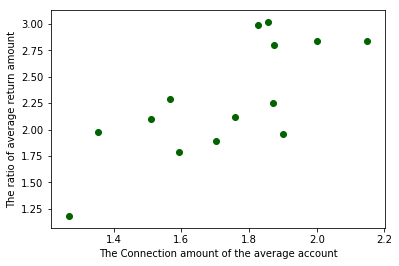### 相关系数R

rDf = examDf.corr()#查看数据间的相关系数
print(rDf)

             Call   Connect    Return
Call     1.000000  0.445870  0.441673
Connect  0.445870  1.000000  0.745338
Return   0.441673  0.745338  1.000000

Connect（接通量）和Return（回款量）的相关系数为0.745338，还不错。

0~0.3 弱相关

0.3~0.6  中等程度相关

0.6~1  强相关

R(相关系数) = X和Y的协方差 / (X的标准差 * Y的标准差) == cov（X,Y）/ σX * σY （即person系数）

### 拆分训练集和测试集

Connect（接通量）和Return（回款量）属于强相关，可以进行线性回归训练，那么我们先来拆分训练集和测试集吧。

#拆分训练集和测试集（train_test_split是存在与sklearn中的函数）
X_train,X_test,Y_train,Y_test = train_test_split(examDf.Connect,examDf.Return,train_size=0.8)
#train为训练数据,test为测试数据,examDf为源数据,train_size 规定了训练数据的占比

print("自变量---源数据:",examDf.Connect.shape, "；  训练集:",X_train.shape, "；  测试集:",X_test.shape)
print("因变量---源数据:",examDf.Return.shape, "；  训练集:",Y_train.shape, "；  测试集:",Y_test.shape)

#散点图
plt.scatter(X_train, Y_train, color="darkgreen", label="train data")#训练集为深绿色点
plt.scatter(X_test, Y_test, color="red", label="test data")#测试集为红色点

#添加标签
plt.legend(loc=2)#图标位于左上角，即第2象限，类似的，1为右上角，3为左下角，4为右下角
plt.xlabel("The Connection amount of the average account")#添加 X 轴名称
plt.ylabel("The ratio of average return amount")#添加 Y 轴名称
plt.show()#显示散点图

自变量---源数据: (14,) ；  训练集: (11,) ；  测试集: (3,)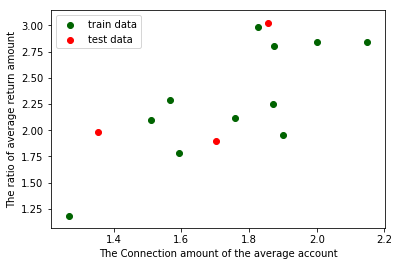#调用线性规划包
model = LinearRegression()

#线性回归训练
model.fit(X_train,Y_train)#调用线性回归包

a  = model.intercept_#截距
b = model.coef_#回归系数

#训练数据的预测值
y_train_pred = model.predict(X_train)
#绘制最佳拟合线：标签用的是训练数据的预测值y_train_pred
plt.plot(X_train, y_train_pred, color='blue', linewidth=2, label="best line")

#测试数据散点图
plt.scatter(X_train, Y_train, color='darkgreen', label="train data")
plt.scatter(X_test, Y_test, color='red', label="test data")

#添加图标标签
plt.legend(loc=2)#图标位于左上角，即第2象限，类似的，1为右上角，3为左下角，4为右下角
plt.xlabel("The Connection amount of the average account")#添加 X 轴名称
plt.ylabel("The ratio of average return amount")#添加 Y 轴名称
plt.show()#显示图像

print("拟合参数:截距",a,",回归系数：",b)
print("最佳拟合线: Y = ",round(a,2),"+",round(b,2),"* X")#显示线性方程，并限制参数的小数位为两位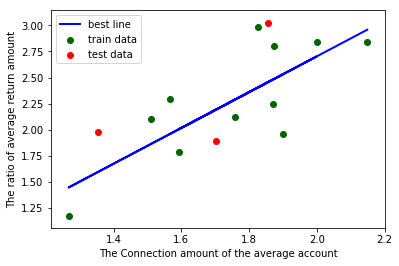拟合参数:截距 -0.7291766860887745 ,回归系数： [1.71584366]


#调用线性规划包
model = LinearRegression()

#在这里加一段
X_train = X_train.values.reshape(-1,1)
X_test = X_test.values.reshape(-1,1)

#线性回归训练
model.fit(X_train,Y_train)#调用线性回归包

a  = model.intercept_#截距
b = model.coef_#回归系数

# 多变量线性回归

### 数据检验（判断是否可以做线性回归）

#-*- coding:utf-8 -*-

import pandas as pd
import numpy as np
import seaborn as sns
import matplotlib.pyplot as plt
from pandas import DataFrame,Series
from sklearn.cross_validation import train_test_split
from sklearn.linear_model import LinearRegression

#读取文件
datafile = u'E:\\pythondata\\dhdhdh.xlsx'#文件所在位置，u为防止路径中有中文名称，此处没有，可以省略
examDf = DataFrame(data)

#数据清洗,比如第一列有可能是日期，这样的话我们就只需要从第二列开始的数据，
#这个情况下，把下面中括号中的0改为1就好，要哪些列取哪些列
new_examDf = examDf.ix[:,0:]

#检验数据
print(new_examDf.describe())#数据描述，会显示最值，平均数等信息，可以简单判断数据中是否有异常值
print(new_examDf[new_examDf.isnull()==True].count())#检验缺失值，若输出为0，说明该列没有缺失值

#输出相关系数，判断是否值得做线性回归模型
print(new_examDf.corr())#0-0.3弱相关；0.3-0.6中相关；0.6-1强相关；

#通过seaborn添加一条最佳拟合直线和95%的置信带，直观判断相关关系
sns.pairplot(data, x_vars=['Call','Connect'], y_vars='Return', size=7, aspect=0.8, kind='reg')
plt.show()


            Call    Connect     Return
count  99.000000  99.000000  99.000000
mean    3.153649   1.967779   2.591121
std     1.027607   0.470364   0.790172
min     1.280100   1.014208   0.941292
25%     2.373528   1.596610   2.044147
50%     3.040000   1.913472   2.476091
75%     3.835980   2.253994   3.035603
max     5.851181   3.234977   5.160840
Call       0
Connect    0
Return     0
dtype: int64
Call   Connect    Return
Call     1.000000  0.837052  0.744792
Connect  0.837052  1.000000  0.844640
Return   0.744792  0.844640  1.000000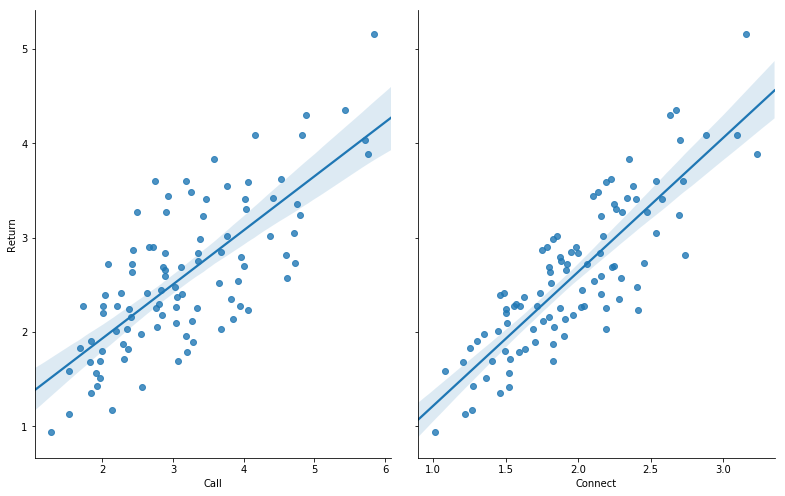• 异常值：通过最值或者平均数或者中位数等判断，或者直接通过查看是否有游离在大部队之外的点来判断是否有异常值；
• 空值：这个没办法，你必须看运行结果的10-12行是否等于0，是0则无空值，如果不是0，就要看看是删掉空值呢？还是用其他值代替呢？不同情况不同对待；
• 相关性：要么通过相关系数的大小判断，要么看散点图中的最佳拟合直线和95%的置信带，直观判断相关关系；

### 训练线性回归模型

#拆分训练集和测试集
X_train,X_test,Y_train,Y_test = train_test_split(new_examDf.ix[:,:2],new_examDf.Return,train_size=0.8)
#new_examDf.ix[:,:2]取了数据中的前两列为自变量，此处与单变量的不同

print("自变量---源数据:",new_examDf.ix[:,:2].shape, "；  训练集:",X_train.shape, "；  测试集:",X_test.shape)
print("因变量---源数据:",examDf.Return.shape, "；  训练集:",Y_train.shape, "；  测试集:",Y_test.shape)

#调用线性规划包
model = LinearRegression()

model.fit(X_train,Y_train)#线性回归训练

a  = model.intercept_#截距
b = model.coef_#回归系数
print("拟合参数:截距",a,",回归系数：",b)

#显示线性方程，并限制参数的小数位为两位
print("最佳拟合线: Y = ",round(a,2),"+",round(b,2),"* X1 + ",round(b,2),"* X2")

Y_pred = model.predict(X_test)#对测试集数据，用predict函数预测

plt.plot(range(len(Y_pred)),Y_pred,'red', linewidth=2.5,label="predict data")
plt.plot(range(len(Y_test)),Y_test,'green',label="test data")
plt.legend(loc=2)
plt.show()#显示预测值与测试值曲线


自变量---源数据: (99, 2) ；  训练集: (79, 2) ；  测试集: (20, 2)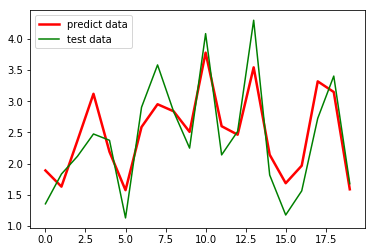12-20

04-214113
09-18
04-272万+
12-11358
08-252万+
01-1023万+
11-231982
09-113326
02-151619
04-109871
04-12
12-12581
09-301612
09-07123
04-1217万+
08-083万+
03-243649

### “相关推荐”对你有帮助么？

•非常没帮助
•没帮助
•一般
•有帮助
•非常有帮助¥2 ¥4 ¥6 ¥10 ¥20余额支付 (余额：-- )扫码支付获取中扫码支付点击重新获取扫码支付1.余额是钱包充值的虚拟货币，按照1:1的比例进行支付金额的抵扣。
2.余额无法直接购买下载，可以购买VIP、C币套餐、付费专栏及课程。余额充值